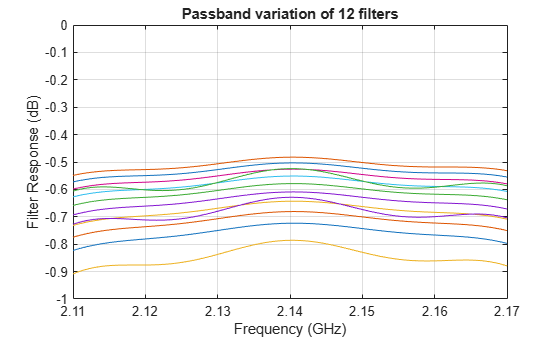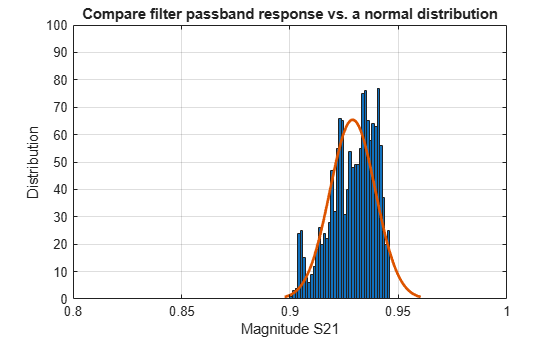## Data Analysis on a Set of S-parameters for an RF Filter

This example shows how to perform statistical analysis on a set of S-parameter data files. First, read twelve S-parameter files representing twelve similar RF filters into the MATLAB workspace and plot them. Next, plot and analyze the passband response of these filters to ensure they meet statistical norms.

### Read in S-parameters from Filter Data Files

Use built-in RF Toolbox functions for reading a set of S-Parameter data files. For each filter, collect and plot the S21 raw values and S21 dB values. The names of the files are AWS_Filter_1.s2p through AWS_Filter_12.s2p and are located in the directory: MATLABROOT/toolbox/rf/rfdemos. These files represent 12 passband filters with similar specifications.

```numfiles = 12; numfreq = 801; % Number of frequency points per file for n = 1:numfiles filename = ['AWS_Filter_',num2str(n),'.s2p']; S = sparameters(filename); s21 = rfparam(S,2,1); s21_data(:,n) = s21; s21_db(:,n) = 20*log10(abs(s21)); end freq = S.Frequencies; % Frequency values are the same for all files figure plot(freq/1e9,s21_db) xlabel('Frequency (GHz)') ylabel('Filter Response (dB)') title('Transmission performance of 12 filters') axis on grid on ```### Filter Passband Visualization

In this section, find, store and plot the S21 data from just the AWS downlink band (2.11 to 2.17 GHz).

```idx = find((freq >= 2.11e9) & (freq <= 2.17e9)); freq_pass_ghz = freq(idx)/1e9; for n = 1:numfiles s21_pass_db(:,n) = s21_db(idx,n); s21_pass_data(:,n) = s21_data(idx,n); end plot(freq_pass_ghz,s21_pass_db) xlabel('Frequency (GHz)') ylabel('Filter Response (dB)') title('Passband variation of 12 filters') axis([min(freq_pass_ghz) max(freq_pass_ghz) -1 0]) grid on ```### Basic Statistical Analysis of the S21 Data

Perform Statistical analysis on the magnitude and phase of all passband S21 data sets. This determines if the data follows a normal distribution and if there is outlier data.

```% Calculate the mean and standard deviation of the magnitude of the entire % passband S21 data set. mean_abs_S21 = mean(abs(s21_pass_data(:))) std_abs_S21 = std(abs(s21_pass_data(:))) % Calculate the mean and standard deviation of the passband magnitude % response at each frequency point. This determines if the data follows a % normal distribution. mean_abs_S21_freq = mean(abs(s21_pass_data),2); std_abs_S21_freq = std(abs(s21_pass_data),0,2); ```
```mean_abs_S21 = 0.9289 std_abs_S21 = 0.0104 ```

Plot all the raw passband magnitude data as a function of frequency, as well as the upper and lower limits defined by the basic statistical analysis.

```hold on plot(freq_pass_ghz,mean_abs_S21_freq) plot(freq_pass_ghz,mean_abs_S21_freq + 2*std_abs_S21_freq,'r') plot(freq_pass_ghz,mean_abs_S21_freq - 2*std_abs_S21_freq,'k') legend('Mean','Mean + 2*STD','Mean - 2*STD') plot(freq_pass_ghz,abs(s21_pass_data),'c','HandleVisibility','off') grid on axis([min(freq_pass_ghz) max(freq_pass_ghz) 0.9 1]); ylabel('Magnitude S21') xlabel('Frequency (GHz)') title('S21 (Magnitude) - Statistical Analysis') hold off ```Plot a histogram for the passband magnitude data. This determines if the upper and lower limits of the data follow a normal distribution.

```histfit(abs(s21_pass_data(:))) grid on axis([0.8 1 0 100]) xlabel('Magnitude S21') ylabel('Distribution') title('Compare filter passband response vs. a normal distribution') ```Calculate the phase response of the passband S21 data, then the per-frequency mean and standard deviation of the phase response. All the passband S21 phase data is then collected into a single vector for later analysis.

```pha_s21 = angle(s21_pass_data)*180/pi; mean_pha_S21 = mean(pha_s21,2); std_pha_S21 = std(pha_s21,0,2); all_pha_data = reshape(pha_s21.',numel(pha_s21),1); % Plot all the raw passband phase data as a function of frequency, as well % as the upper and lower limits defined by the basic statistical analysis. hold on plot(freq_pass_ghz,mean_pha_S21) plot(freq_pass_ghz,mean_pha_S21 + 2*std_pha_S21,'r') plot(freq_pass_ghz,mean_pha_S21 - 2*std_pha_S21,'k') legend('Mean','Mean + 2*STD','Mean - 2*STD') plot(freq_pass_ghz,pha_s21,'c','HandleVisibility','off') grid on axis([min(freq_pass_ghz) max(freq_pass_ghz) -180 180]) ylabel('Phase S21') xlabel('Frequency (GHz)') title('S21 (Phase) - Statistical Analysis') hold off ```Plot a histogram for the passband phase data. This determines if the upper and lower limits of the data follow a uniform distribution.

```histogram(all_pha_data,35) grid on axis([-180 180 0 100]) xlabel('Phase S21 (degrees)') ylabel('Distribution') title('Histogram of the filter phase response') ```### ANOVA Analysis of the S21 Data

```% Perform ANOVA analysis on both the magnitude and phase passband data. anova1(abs(s21_pass_data).',freq_pass_ghz); ylabel('Magnitude S21') xlabel('Frequency (GHz)') ax1 = gca; ax1.XTick = 0.5:10:120.5; ax1.XTickLabel = {2.11,'',2.12,'',2.13,'',2.14,'',2.15,'',2.16,'',2.17}; title('Analysis of variance (ANOVA) of passband loss') grid on ``````anova1(pha_s21.',freq_pass_ghz); ylabel('Phase S21 (degrees)') xlabel('Frequency (GHz)') ax2 = gca; ax2.XTick = 0.5:10:120.5; ax2.XTickLabel = {2.11,'',2.12,'',2.13,'',2.14,'',2.15,'',2.16,'',2.17}; title('Analysis of variance (ANOVA) of passband phase response') grid on ```### Fit the Phase Data to 1st-Order Polynomial

```% Perform a curve fit of the S21 phase data using a linear regression % model. phase_s21_fit = fit(repmat(freq_pass_ghz,numfiles,1),all_pha_data,'poly1') ```
```phase_s21_fit = Linear model Poly1: phase_s21_fit(x) = p1*x + p2 Coefficients (with 95% confidence bounds): p1 = -310.8 (-500.9, -120.6) p2 = 665.3 (258.3, 1072) ```